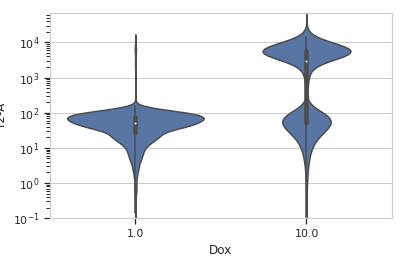# cytoflow.views.violin¶

class cytoflow.views.violin.ViolinPlotView[source]

Plots a violin plot – a facetted set of kernel density estimates.

variable

the main variable by which we’re faceting

Type: Str
channel

The channel to view

Type: Str
scale

The scale applied to the data before plotting it.

Type: {‘linear’, ‘log’, ‘logicle’}
xfacet, yfacet

Set to one of the conditions in the Experiment, and a new row or column of subplots will be added for every unique value of that condition.

Type: String
huefacet

Set to one of the conditions in the in the Experiment, and a new color will be added to the plot for every unique value of that condition.

Type: String
huescale

How should the color scale for huefacet be scaled?

Type: {‘linear’, ‘log’, ‘logicle’}

Examples

Make a little data set.

>>> import cytoflow as flow
>>> import_op = flow.ImportOp()
>>> import_op.tubes = [flow.Tube(file = "Plate01/RFP_Well_A3.fcs",
...                              conditions = {'Dox' : 10.0}),
...                    flow.Tube(file = "Plate01/CFP_Well_A4.fcs",
...                              conditions = {'Dox' : 1.0})]
>>> import_op.conditions = {'Dox' : 'float'}
>>> ex = import_op.apply()


Plot a violin plot

>>> flow.ViolinPlotView(channel = 'Y2-A',
...                     scale = 'log',
...                     variable = 'Dox').plot(ex)plot(experiment, **kwargs)[source]

Plot a violin plot of a variable

Parameters: experiment (Experiment) – The Experiment to plot using this view. title (str) – Set the plot title xlabel, ylabel (str) – Set the X and Y axis labels huelabel (str) – Set the label for the hue facet (in the legend) legend (bool) – Plot a legend for the color or hue facet? Defaults to True. sharex, sharey (bool) – If there are multiple subplots, should they share axes? Defaults to True. row_order, col_order, hue_order (list) – Override the row/column/hue facet value order with the given list. If a value is not given in the ordering, it is not plotted. Defaults to a “natural ordering” of all the values. height (float) – The height of each row in inches. Default = 3.0 aspect (float) – The aspect ratio of each subplot. Default = 1.5 col_wrap (int) – If xfacet is set and yfacet is not set, you can “wrap” the subplots around so that they form a multi-row grid by setting col_wrap to the number of columns you want. sns_style ({“darkgrid”, “whitegrid”, “dark”, “white”, “ticks”}) – Which seaborn style to apply to the plot? Default is whitegrid. sns_context ({“paper”, “notebook”, “talk”, “poster”}) – Which seaborn context to use? Controls the scaling of plot elements such as tick labels and the legend. Default is talk. despine (Bool) – Remove the top and right axes from the plot? Default is True. min_quantile (float (>0.0 and <1.0, default = 0.001)) – Clip data that is less than this quantile. max_quantile (float (>0.0 and <1.0, default = 1.00)) – Clip data that is greater than this quantile. lim ((float, float)) – Set the range of the plot’s data axis. orientation ({‘vertical’, ‘horizontal’}) bw ({{‘scott’, ‘silverman’, float}}, optional) – Either the name of a reference rule or the scale factor to use when computing the kernel bandwidth. The actual kernel size will be determined by multiplying the scale factor by the standard deviation of the data within each bin. scale_plot ({{“area”, “count”, “width”}}, optional) – The method used to scale the width of each violin. If area, each violin will have the same area. If count, the width of the violins will be scaled by the number of observations in that bin. If width, each violin will have the same width. scale_hue (bool, optional) – When nesting violins using a hue variable, this parameter determines whether the scaling is computed within each level of the major grouping variable (scale_hue=True) or across all the violins on the plot (scale_hue=False). gridsize (int, optional) – Number of points in the discrete grid used to compute the kernel density estimate. inner ({{“box”, “quartile”, None}}, optional) – Representation of the datapoints in the violin interior. If box, draw a miniature boxplot. If quartiles, draw the quartiles of the distribution. If point or stick, show each underlying datapoint. Using None will draw unadorned violins. split (bool, optional) – When using hue nesting with a variable that takes two levels, setting split to True will draw half of a violin for each level. This can make it easier to directly compare the distributions.